### Home > CAAC > Chapter 8 > Lesson 8.3.3 > Problem8-119

8-119.
1. Solve the equations below for x. Check your solutions. Homework Help ✎

1. 3x2 + 3x = 6 + 3x2

2.3. 5 − (2x −3) = −3x + 6

4. 6(x − 3) + 2x = 4(2x + 1) − 22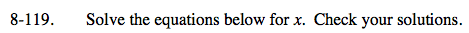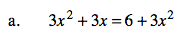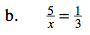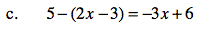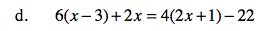One of the solutions is all real numbers. You should be able to check your solutions to the rest of the equations.